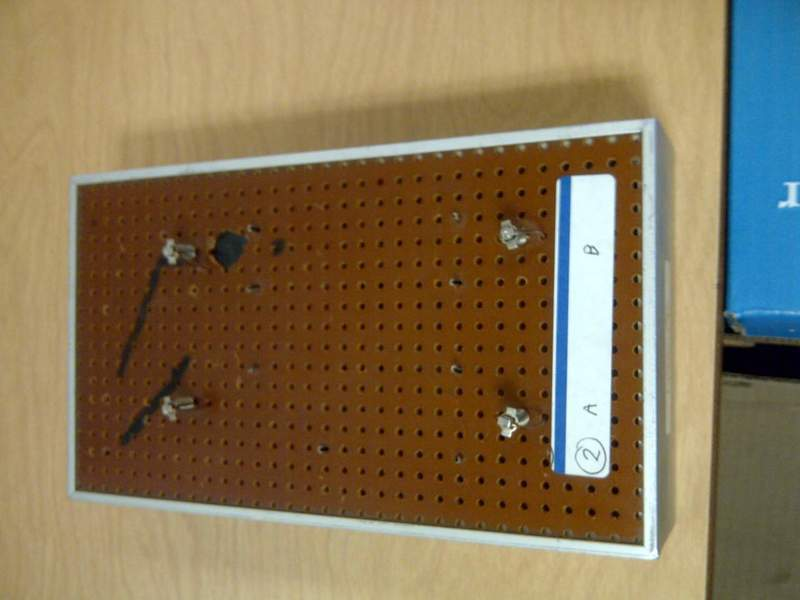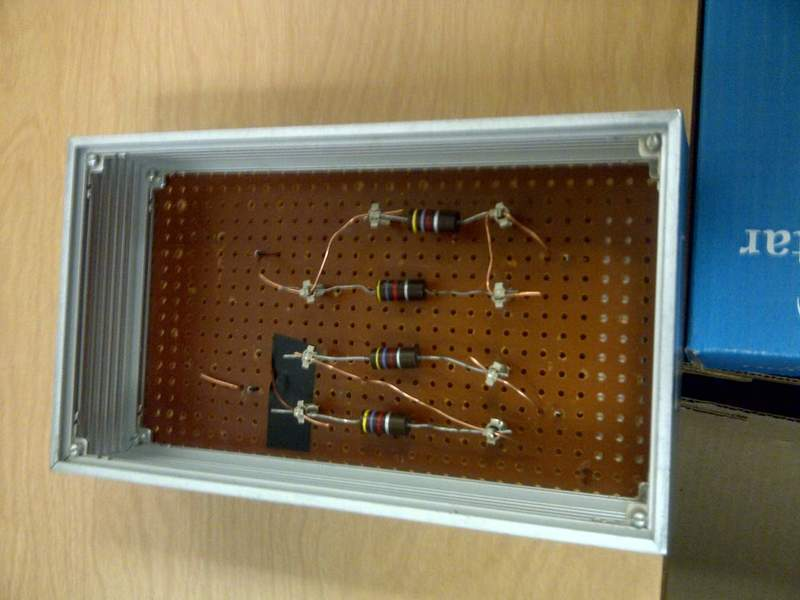# Finding Which Resistance and Which Circuit is Series and Which is Parallel

## Homework Statement

I need to design a lab to solve for which circuit is parallel, and which is series. I also need to figure out the resistance in each resistor (each resistor is the same, so I need to find one of the resistances).

Here is the information given:
Given four identical resistors and two circuits, one in parallel, one in series, and both including two of the four resistors, which circuit is parallel, which is series, and what is the resistance of each resistor.

## Homework Equations

V=IR
Series Circuit: RT=R1+R2+R3+...
Parallel Circuit: 1/RT=1/R1+1/R2+1/R3+...

## The Attempt at a Solution

In the series circuit:
V = IR
R = V/I

Rresistor = R/2 (since the resistance of each resistor is the same)

The two things that I haven't been able to figure out are how I can figure out...
1. Which circuit is which (either parallel or series)
2. The resistance of the parallel circuit.

P.S. This is all DC current.

Thanks for your help! Any ideas are appreciated!

Here is a picture of the circuit apparatus, in the real lab, the box will be sealed and it will not be known which circuit is parallel and which is series.gneill
Mentor
Try a couple of sample values for the resistance and calculate the expected resistance you would measure for each case.

Presumably in the lab you'll be measuring the resistance of the hidden circuits and then be asked to identify which is which. The above exercise should help you see what to look for.

One little tip:
When you have resistors in parallel.... it does not matter how many.... the combined resistance is ALWAYS less than the smallest resistor in the combination.
eg 10 + 4 +100 +6 +20 + 2 +9 + 4 in parallel must be less than 2
Not much... but I find it useful and great for catching students out!!

One little tip:
When you have resistors in parallel.... it does not matter how many.... the combined resistance is ALWAYS less than the smallest resistor in the combination.
eg 10 + 4 +100 +6 +20 + 2 +9 + 4 in parallel must be less than 2
Not much... but I find it useful and great for catching students out!!